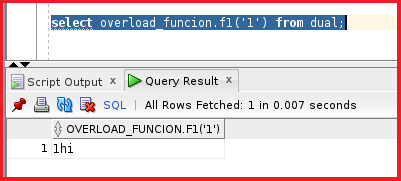##### Method 1: Creating Package Specification

Execute the below statements to Create the package specification.

```create or replace package overload_funcion
is
function f1 (p1 number) return number;
function f1 (p1 number) return varchar2;
end;
```

This is the package specification named overload_funcion which has two functions in it. The function f1 takes p1 as input and returns a number and the function f2 takes p1 as input and returns varchar2. We are trying to understand whether Oracle is able to understand which function needs to choose while calling the function through the package. Now, let’s try to compile this package specification.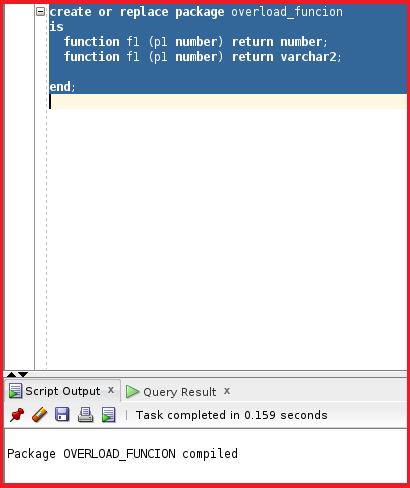So, the package specification is compiled. Let’s try to look at the package body as follows.

```create or replace package body overload_funcion
is
function f1 (p1 number) return number
is
begin
return p1;
end;

function f1 (p1 number) return varchar2
is
begin
return p1||'hi';
end;

end;
```

The package body code consists of two functions. The function f1 takes p1 as input and returns the same p1 parameter as the output with the same data type. The function f2 takes the p1 as input and returns varchar2 which concatenates with p1. This is the simple code. Let’s try to compile this package body.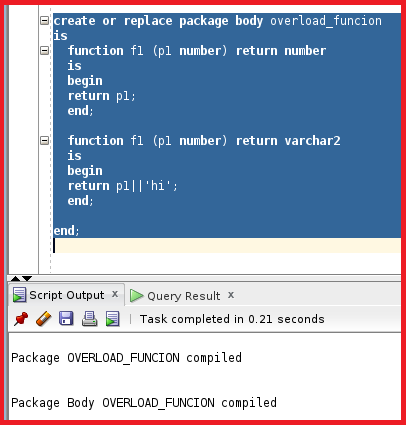So, the package body is also compiled now. Let’s try to test this function by executing the function. These two functions are of the same name taking p1 as the input parameter and returning a different datatype as the output. Oracle cannot understand because the functions with the same input parameters and cannot distinguish between two functions. So, execute the below select statement.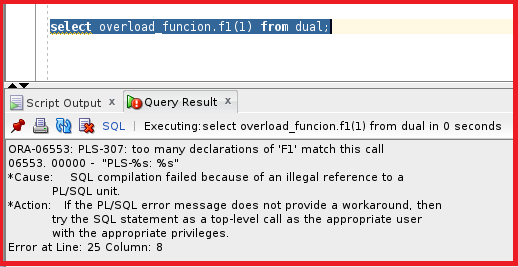We can see the error showing “ too many declarations of F1 match this call”. So, the oracle cannot distinguish between two functions.

##### Method 2:

In this case, the functions take the same parameters and datatypes as input and return the same datatype as output.

```create or replace package overload_funcion
is
function f1 (p1 number) return number;
function f1 (p1 varchar2) return varchar2;

end;
```

So, we can see the function f1 takes input as p1 which is a number, and returns a number and, in the function, f1 takes p1 as varchar2 and returns varchar2. Let’s try to compile the package specification.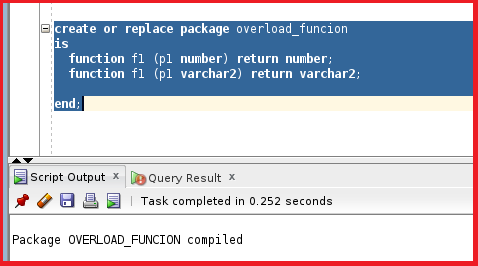The package specification is compiled. Let’s see the package body. The package body takes p1 with datatype as number and returns number and the other function takes f1 as input with datatype as varchar2 and returns varchar2.

```create or replace package body overload_funcion
is
function f1 (p1 number) return number
is
begin
return p1;
end;

function f1 (p1 varchar2) return varchar2
is
begin
return p1||'hi';
end;

end;
```

Let’s compile the package body and see how this works.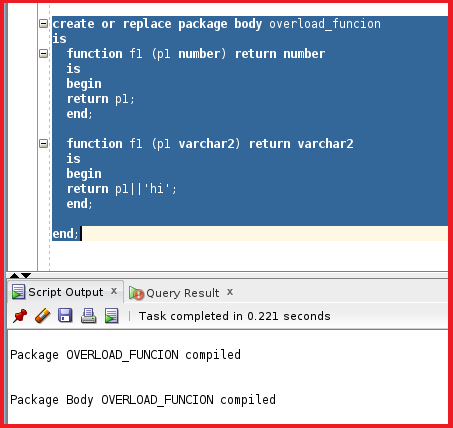So, the package body is now compiled. Let’s now try to test this package by executing the package.

Let’s try to run this statement which calls the function f1 with the number datatype.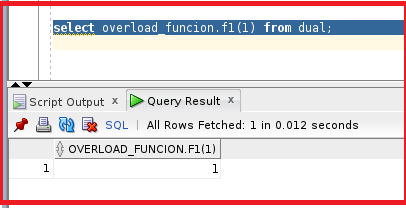So, the function is working fine while calling with the number datatype. Let’s now try to call with varchar2 datatype and see how it works.